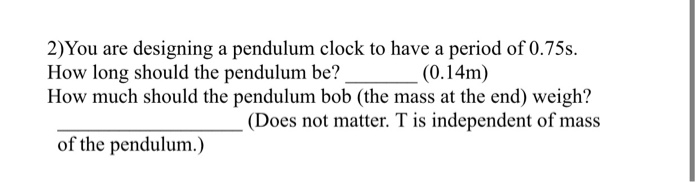# 2) You are designing a pendulum clock to have a period of 0.75s. How long should...

###### Question:2) You are designing a pendulum clock to have a period of 0.75s. How long should the pendulum be? (0.14m) How much should the pendulum bob (the mass at the end) weigh? (Does not matter. T is independent of mass of the pendulum.)